M358K First Midterm Exam (with solutions)
Given October 3, 2001

1. True or false. (2 pages) If the statement is false, explain why (e.g., give an example where the statement fails).

a) The best way to display data on a single variable is with a stem-and-leaf plot.

FALSE. Stem-and-leaf plots are great for handling moderate amounts of data, but you'd never use one for 50,000 data points. More likely you'd use a histogram.

b) If the mean and median of a distribution are the same, then the distribution is symmetric.

FALSE. As an example, the data set { 0, 0, 1, 1, 3} has mean and median equal to 1, but is not symmetric.

c) The mean and standard deviation of a variable are sensitive to the values of a few outliers, while the median and inter-quartile range are not.

TRUE.

d) If the correlation between x and y is 0.56, then an increase of x by one standard deviation is associated, on average, with y increasing by 0.56 standard deviations.

TRUE.

e) If the least-squares regression line for y in terms of x is y = x +4, then the least-squares regression line for x in terms of y is x = y-4.

FALSE (unless r=1). The slope of the ``x in terms of y'' regression line is not 1 over the slope of the ``y in terms of x'' line. Rather, it is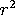over the slope of the ``y in terms of x'' line.

f) The slope of a least-squares regression line is the same as the correlation coefficient.

FALSE. Slope =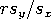. Even without the formula, note that the slope is dimensionfull (units of y / units of x), while the correlation coefficient is dimensionless.

g) If the correlation between x and y is +1, then x causes y.

FALSE. Correlation is not causation.

h) In a random sample, each element of the population has an equal chance of being selected.

TRUE.

i) When sampling a large population, you need a bigger sample than when sampling a small population.

FALSE. The accuracy of a sample depends on the size of the sample, not the size of the population.

j) A well-designed experiment requires two or more groups of test subjects.

TRUE. You always need a control group.

2. Mindless calculation:

Suppose we have two variables x and y, with x the explanatory variable and y the response variable. You measure the five data points (x,y) = (1,-5), (2,-1), (3,0), (4,3) and (5,3).

a) Compute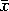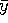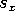, and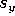.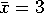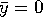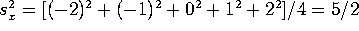, so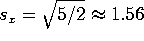.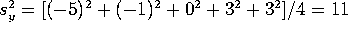, so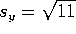.

b) What is the correlation coefficient between x and y?

Cov(x,y) = [(-2)(-5)+(-1)(-1)+ 0 + 1(3) + 2(3)]/4 = 5.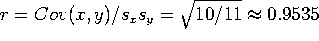c) What is the equation of the least-squares regression line?

Slope =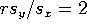(exactly). Goes through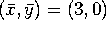, so equation is y-0 = 2(x-3), or y= 2x-6.

d) What fraction of the variation in y is explained by variation in x?

Fraction explained by x is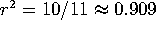.

3. Displaying data:

A variable X takes on the values 3, 15, 24, 39, 27, 34, 12, 23, 27, 41, 18, 19, 33 and 51.

a) Display this data in a stem-and-leaf plot.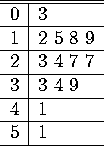b) Which points would you consider to be outliers? Why?

Note that the median is 25.5 (halfway between 24 and 27), Q1=18 and Q3=34, so the IQR is 16. I would call 3 and 51 outliers, since they're the only points far above Q3 or far below Q1. There's a 9 or 10 point gap between these points and the nearest other points. However, other answers are also reasonable. I'll be grading largely on how well you justify whatever answer you put down.

c) If you had to boil this data down to a few numbers, would you use the mean and standard deviation or the max, min, median, Q1 and Q3? Why?

Since the distribution is fairly symmetric and reasonably bell-shaped, either will do. However, I personally would prefer 5-point data.

d) Draw a box plot (not a box-and-whiskers plot) for the distribution.

Sorry, but I can't draw this online. However, the box should go from Q1=18 to Q3=34, with a line at M=25.5, and should show the points that lie outside the box, namely 3, 12, 15, 39, 41 and 51.

4. Normal distributions

a) In reality (if one can use that word for a made-up problem), ``3 inch'' Acme Widgets are not exactly 3 inches long. Their length is given by a normal distribution with a mean of 3.05 inches and a standard deviation of .05 inches. To work properly, a widget must be between 2.95 and 3.2 inches long. What fraction of Acme's product are defective?

3.2 inches is 3 standard deviations above average, while 2.95 inches is 2 standard deviations below average. The probability of a widget being good is F(3) - F(-2) = 0.9987 - 0.0228 = 0.9759. The probability of it NOT being good is 0.0241 = 2.41%.

b) The weight of bread loaves that comes out of a certain machine is normally distributed, with the mean determined by the settings on the machine and a standard deviation of 0.5 ounces. If you want to make sure that 99% of the loaves have a weight of 16 ounces or more, how should you set the machine?

Since F(2.33)=0.99, you need a cushion of 2.33 standard deviations, so set the machine for a mean of 16 + 2.33(0.5) = 17.16 ounces.